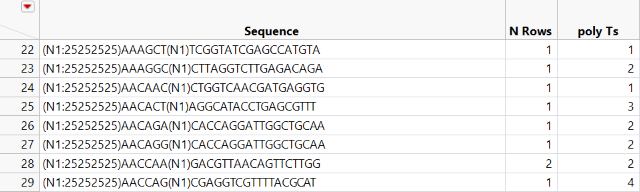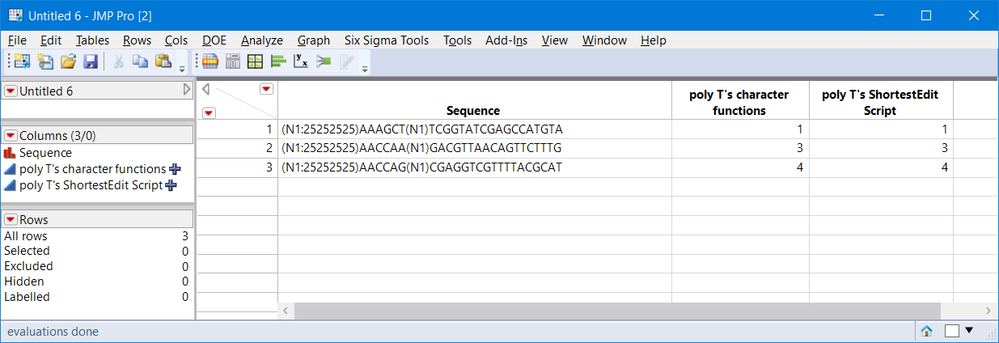Choose Language Hide Translation Bar
Highlighted

## Count the number of Ts in a sequence

Hello,

I am trying to find a formula that will count highest number times a letter is repeated consecuteivley in a sequence. I have attached an example where I am trying to write formula for poly Ts column, and it will generate count of Ts in a sequence consectively.Thank you,

Pratish

1 ACCEPTED SOLUTION

Accepted Solutions
Highlighted

## Re: Count the number of Ts in a sequence

A regular expression expert might have a nice pattern to scan and find all matches, but that is beyond my REGEX skills.

I have provided two solutions that can be done using column formulas. Both might need some explanation.

The first uses nested character functions, the second uses the ShortestEditScript() function. By the way, you did not specify if you are counting T sequences prior to (N1), both use the entire string. The example table is attached and explanations are belowAssume    s2 = "(N1:25252525)AACCAA(N1)GACGTTAACAGTTCTTTG";

Character functions: Words(), Sort List(), Reverse(), list[n], Length()

``Length(Reverse(Sort List(words(s2,"ACGN():0123456789"))));``

Here is the log output for the respective functions

``````//:*/
words(s2,"ACGN():0123456789")
/*:
{"TT", "TT", "TTT"}
//:*/
Reverse(Sort List(words(s2,"ACGN():0123456789")))
/*:
{"TTT", "TT", "TT"}
//:*/
Reverse(Sort List(words(s2,"ACGN():0123456789")))
/*:
"TTT"
//:*/
Length(Reverse(Sort List(words(s2,"ACGN():0123456789"))));
/*:
3``````

ShortestEditScript() is an interesting function. Script 9_Extra_ShortestEditDistance.jsl written for JSL Companion, Applications of the JMP Scripting Language Second Edition document 4 different methods for using this powerful and useful function.  For this example, I am using Sequnces() and requesting matrix output. It would take too much space to document this completely, in this forum, so I will just show the results and add a few comments.

`````` msed = Shortest Edit Script( Strings(s2, Repeat("T",length(s2)),matrix(1)) );
maximum(msed[loc(msed[0,1]==0),4]);``````

The two strings being compared are s2 and a string of all T's created by function Repeat("T", length(s2) ).

`````` msed = Shortest Edit Script( Strings(s2, Repeat("T",length(s2)),matrix(1)) );

/*:
[-1 1 . 27,
0 28 1 2,
-1 30 . 5,
0 35 3 2,
-1 37 . 1,
0 38 5 3,
-1 41 . 1,
1 . 8 34]

/* The matrix output  n x 4 where n = nrow(msed)
Column1:  -1 | 1| 0  -1-->remove, 1-->insert, 0-->common
Column4:  length
*/``````

So now it is a matter of finding the locations in the 1st column of the matrix with 0's (matches/common/T's) this can be done with the loc() function. Then the length of the matching sequence is in the 4th column, so just find the maximum.  Note msed[0,1] represents the 1st column of the matrix msed.

``loc(msed[0,1]==0)/*:[2, 4, 6] //:*/msed[loc(msed[0,1]==0),4]/*:[2, 2, 3]//:*/ maximum(msed[loc(msed[0,1]==0),4]);/*:3``

It will be interesting to see other solutions.

4 REPLIES 4
Highlighted

## Re: Count the number of Ts in a sequence

A regular expression expert might have a nice pattern to scan and find all matches, but that is beyond my REGEX skills.

I have provided two solutions that can be done using column formulas. Both might need some explanation.

The first uses nested character functions, the second uses the ShortestEditScript() function. By the way, you did not specify if you are counting T sequences prior to (N1), both use the entire string. The example table is attached and explanations are belowAssume    s2 = "(N1:25252525)AACCAA(N1)GACGTTAACAGTTCTTTG";

Character functions: Words(), Sort List(), Reverse(), list[n], Length()

``Length(Reverse(Sort List(words(s2,"ACGN():0123456789"))));``

Here is the log output for the respective functions

``````//:*/
words(s2,"ACGN():0123456789")
/*:
{"TT", "TT", "TTT"}
//:*/
Reverse(Sort List(words(s2,"ACGN():0123456789")))
/*:
{"TTT", "TT", "TT"}
//:*/
Reverse(Sort List(words(s2,"ACGN():0123456789")))
/*:
"TTT"
//:*/
Length(Reverse(Sort List(words(s2,"ACGN():0123456789"))));
/*:
3``````

ShortestEditScript() is an interesting function. Script 9_Extra_ShortestEditDistance.jsl written for JSL Companion, Applications of the JMP Scripting Language Second Edition document 4 different methods for using this powerful and useful function.  For this example, I am using Sequnces() and requesting matrix output. It would take too much space to document this completely, in this forum, so I will just show the results and add a few comments.

`````` msed = Shortest Edit Script( Strings(s2, Repeat("T",length(s2)),matrix(1)) );
maximum(msed[loc(msed[0,1]==0),4]);``````

The two strings being compared are s2 and a string of all T's created by function Repeat("T", length(s2) ).

`````` msed = Shortest Edit Script( Strings(s2, Repeat("T",length(s2)),matrix(1)) );

/*:
[-1 1 . 27,
0 28 1 2,
-1 30 . 5,
0 35 3 2,
-1 37 . 1,
0 38 5 3,
-1 41 . 1,
1 . 8 34]

/* The matrix output  n x 4 where n = nrow(msed)
Column1:  -1 | 1| 0  -1-->remove, 1-->insert, 0-->common
Column4:  length
*/``````

So now it is a matter of finding the locations in the 1st column of the matrix with 0's (matches/common/T's) this can be done with the loc() function. Then the length of the matching sequence is in the 4th column, so just find the maximum.  Note msed[0,1] represents the 1st column of the matrix msed.

``loc(msed[0,1]==0)/*:[2, 4, 6] //:*/msed[loc(msed[0,1]==0),4]/*:[2, 2, 3]//:*/ maximum(msed[loc(msed[0,1]==0),4]);/*:3``

It will be interesting to see other solutions.

Highlighted

## Re: Count the number of Ts in a sequence

Here's a simple brute force approach; not sure of the performance relative to @gzmorgan0's methods.

``````s2 = "(N1:25252525)AACCAA(N1)GACGTTAACAGTTCTTTG";
len = length(s2);
tstring = repeat("T", len);
maxlen  = 0;
for (i = len, i >= 1, i--,
if (contains(s2, tstring),
maxlen = i;
break();
,
tstring = substr(tstring, 2);
);
);
show(maxlen);``````
Highlighted

## Re: Count the number of Ts in a sequence

In the spirit of 'other solutions' here's another brute force one:

``````NamesDefaultToHere(1);

// Given a string and a single character, finds the longest sequence of that character
// and returns the length and starting position of that sequence. If the sequence
// occurs more than once, only the first is identified
findLongestRepeatedCharacter =
Function({str, char}, {Default Local},
n = Length(str);
count = 0;
currentCount = 1;
// Traverse the string except for the last character
for (i = 1, i <= n-1, i++,
thisChar = Substr(str, i, 1);
nextChar = Substr(str, i+1, 1);
// If the current character and the next are both 'char' ...
if((thisChar == char & nextChar == char),
// ... increment 'currentCount'
currentCount++,
// ... else if they're not ...
if(currentCount > count,
// ... record 'currentCount' if it's bigger than we've seen so far
count = currentCount;
);
// ... and reset 'currentCount'
currentCount = 1;
);
);
// Build the sequence we've found
seq = Repeat(char, count);
// Find where it occurs
pos = Munger(str, 1, seq);
// Return the results
if (pos == 0,
EvalList({0, pos}),
EvalList({count, pos})
);
);

// Try it out
str = "(N1:25252525)AACCAA(N1)GACGTTAACAGTTCTTTG";
Print(findLongestRepeatedCharacter(str, "T"));
Print(findLongestRepeatedCharacter(str, "A"));
Print(findLongestRepeatedCharacter(str, "X"));``````
Highlighted

## Re: Count the number of Ts in a sequence

Table formula using ShortestEditScript() used s2 in a portion of the formula that should have been Sequence. Table with corrected function is attached.

Article Labels

There are no labels assigned to this post.